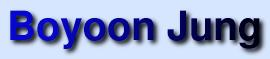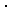Boyoon Jung's Library Manual

bj::SDLWriter Class Reference [Sensor Data Logging]

A data logger into a SDLog file. More...

#include <sdl_writer.h>

Collaboration diagram for bj::SDLWriter:[legend]
List of all members.

Public Member Functions

SDLWriter (char *filename=0, double interval=0.1)
A constructor.
~SDLWriter (void)
A destructor.
bool open (char *filename, double interval=0.1)
Open a log file.
bool close (void)
Close a log file.
bool isOpen (void)
Check if any log file has been open.
void loadGPS (double longitude, double latitude, double altitude, double geoid=0.0f, double dop=0, double timestamp=0.0)
void loadSonar (int size, float ranges[], double timestamp=0.0)
void loadLaser (float from, float to, float resolution, float ranges[], bool dazzles[]=0, double timestamp=0.0)
void loadIMU (double roll, double pitch, double yaw, double timestamp=0.0)
void loadCamera (unsigned long frame, double timestamp=0.0)
void loadStereo (unsigned long frame, double timestamp=0.0)
void loadMotor (double linear, double angular, double timestamp=0.0)
int flush (double timestamp=0.0)
Flush the data buffer.
int dump (sdl_data_t &data, int sensors=SDL_ALL, double timestamp=0.0)
Dump a set of data.

Detailed Description

A data logger into a SDLog file.

SDLWriter class provides a convenient way to dump sensor data into a SDLog file.

Constructor & Destructor Documentation

 bj::SDLWriter::SDLWriter ( char * filename = 0, double interval = 0.1 )
 A constructor.

 bj::SDLWriter::~SDLWriter ( void )
 A destructor.

Member Function Documentation

 bool bj::SDLWriter::close ( void )
 Close a log file.

 int bj::SDLWriter::dump ( sdl_data_t & data, int sensors = SDL_ALL, double timestamp = 0.0 )
 Dump a set of data.

 int bj::SDLWriter::flush ( double timestamp = 0.0 )
 Flush the data buffer.

 bool bj::SDLWriter::isOpen ( void ) [inline]
 Check if any log file has been open.

 void bj::SDLWriter::loadCamera ( unsigned long frame, double timestamp = 0.0 )

 void bj::SDLWriter::loadGPS ( double longitude, double latitude, double altitude, double geoid = 0.0f, double dop = 0, double timestamp = 0.0 )

 void bj::SDLWriter::loadIMU ( double roll, double pitch, double yaw, double timestamp = 0.0 )

 void bj::SDLWriter::loadLaser ( float from, float to, float resolution, float ranges[], bool dazzles[] = 0, double timestamp = 0.0 )

 void bj::SDLWriter::loadMotor ( double linear, double angular, double timestamp = 0.0 )

 void bj::SDLWriter::loadOdometer ( double x, double y, double heading, double timestamp = 0.0 )

 void bj::SDLWriter::loadSonar ( int size, float ranges[], double timestamp = 0.0 )

 void bj::SDLWriter::loadStereo ( unsigned long frame, double timestamp = 0.0 )

 bool bj::SDLWriter::open ( char * filename, double interval = 0.1 )
 Open a log file.

The documentation for this class was generated from the following file:
ResearchProjectsPublications

ToolsBjLib ManualPlayer/StagePyPlayerMicroStrain IMUGPS/DGPSParticle Filter

PersonalWelcomeCurriculum VitaeResumeContactUSCUSC CS DeptCRESRobotics LabRESL